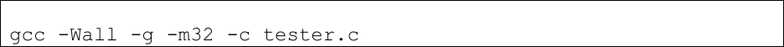Textbook Notes (280,000)
US (110,000)
LSU (20,000)
CSC (200)
All (7)
Chapter

# Project 1

Department
Computer Science
Course Code
CSC 3501
Professor
All

This preview shows half of the first page. to view the full 3 pages of the document.Assignment 1: Bit-level operations
Due: 11:59PM Tuesday, September 29, 2015
In this assignment you will become familiar with doing basic bit-level operations, and get further
practice with Unix-style programming. First, create the directory prog1, which will be used for
submission. Change to this directory, and issue the following command to get the files you need:
cp ~cs3501_lee/cs3501_F15/p1/* .
View and understand the provided Makefile and tester.c so that you can write your own
in the future.
You will write two functions prototyped below in the file int2bitstr.c:
void int2bitstr(int I, char *str);
int get_exp_value(float f);
For the function int2bitstr, I is input, and str is output, and you will write to str a 32-
length string (remember that strings are null-terminated!) of 0s and 1s that indicate the bit pattern
of the 32-bit integer I. The first character in the string will be the most significant bit from I,
and the last non-NULL character will be the least significant. You must use bit-level operations
to determine whether a character should be a zero or one. The only allowed integer arithmetic is
that of the loop itself, which must not do this calculation.
Forbidden operations for int2bitstr:
Switch statements, function calls, and macro invocations.
Division, modulus, and multiplication.
For the function get_exp_value, f is input, and you will calculate and return the exponent
value of f. You may want to use the provided function f2u to get the bit pattern of f. The bias
of float (single precision) is 127.
Forbidden operations for get_exp_value:
Loops, switch statements, function calls (except unsigned f2u(float f)), and
macro invocations.
Division, modulus, and multiplication.
Relative comparison operators (<, >, <=, and >=). You can use Equality (==) and
inequality (!=) tests.
Here is an example run of my completed tester:
>make
gcc -Wall -g -m32 -c tester.c